# Rectifying Diodes

## Discrete Semiconductor Devices and Circuits

• #### Question 1

 Don’t just sit there! Build something!!

Learning to mathematically analyze circuits requires much study and practice. Typically, students practice by working through lots of sample problems and checking their answers against those provided by the textbook or the instructor. While this is good, there is a much better way.

You will learn much more by actually building and analyzing real circuits, letting your test equipment provide the “answers” instead of a book or another person. For successful circuit-building exercises, follow these steps:

1. Carefully measure and record all component values prior to circuit construction, choosing resistor values high enough to make damage to any active components unlikely.
2. Draw the schematic diagram for the circuit to be analyzed.
3. Carefully build this circuit on a breadboard or other convenient medium.
4. Check the accuracy of the circuit’s construction, following each wire to each connection point, and verifying these elements one-by-one on the diagram.
5. Mathematically analyze the circuit, solving for all voltage and current values.
6. Carefully measure all voltages and currents, to verify the accuracy of your analysis.
7. If there are any substantial errors (greater than a few percent), carefully check your circuit’s construction against the diagram, then carefully re-calculate the values and re-measure.

When students are first learning about semiconductor devices, and are most likely to damage them by making improper connections in their circuits, I recommend they experiment with large, high-wattage components (1N4001 rectifying diodes, TO-220 or TO-3 case power transistors, etc.), and using dry-cell battery power sources rather than a benchtop power supply. This decreases the likelihood of component damage.

As usual, avoid very high and very low resistor values, to avoid measurement errors caused by meter “loading” (on the high end) and to avoid transistor burnout (on the low end). I recommend resistors between 1 kΩ and 100 kΩ.

One way you can save time and reduce the possibility of error is to begin with a very simple circuit and incrementally add components to increase its complexity after each analysis, rather than building a whole new circuit for each practice problem. Another time-saving technique is to re-use the same components in a variety of different circuit configurations. This way, you won’t have to measure any component’s value more than once.

• #### Question 2

When plotted on a curve tracer, the characteristic curve for a normal PN junction rectifying diode looks something like this: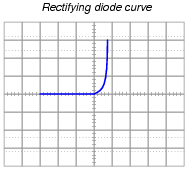Label each axis (horizontal and vertical) of the curve tracer graph, then determine whether the diode behaves more like a voltage source or more like a current source (i.e. does it try to maintain constant voltage or does it try to maintain constant current?) when it is conducting current.

Models are very useful because they simplify circuit approximations. For example, we can analyze this diode circuit quite easily if we substitute an electrical source in place of the diode: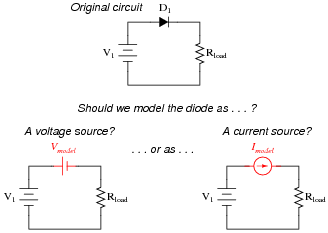The only question here is, which substitution makes the most sense? Based on the diode’s characteristic curve behavior, should we substitute a voltage source or a current source in place of it? Assuming this is a 1N4001 rectifying diode, what is the value we should use for the substituting source?

• #### Question 3

The following schematic diagram is of a simple curve tracer circuit, used to plot the current/voltage characteristics of different electronic components on an oscilloscope screen:The way it works is by applying an AC voltage across the terminals of the device under test, outputting two different voltage signals to the oscilloscope. One signal, driving the horizontal axis of the oscilloscope, represents the voltage across the two terminals of the device. The other signal, driving the vertical axis of the oscilloscope, is the voltage dropped across the shunt resistor, representing current through the device. With the oscilloscope set for “X-Y” mode, the electron beam traces the device’s characteristic curve.

For example, a simple resistor would generate this oscilloscope display: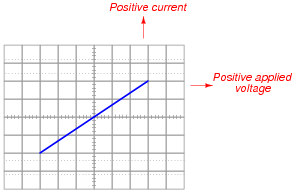A resistor of greater value (more ohms of resistance) would generate a characteristic plot with a shallower slope, representing less current for the same amount of applied voltage: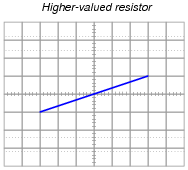Curve tracer circuits find their real value in testing semiconductor components, whose voltage/current behaviors are nonlinear. Take for instance this characteristic curve for an ordinary rectifying diode: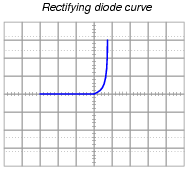The trace is flat everywhere left of center where the applied voltage is negative, indicating no diode current when it is reverse-biased. To the right of center, though, the trace bends sharply upward, indicating exponential diode current with increasing applied voltage (forward-biased) just as the “diode equation” predicts.

On the following grids, plot the characteristic curve for a diode that is failed shorted, and also for one that is failed open: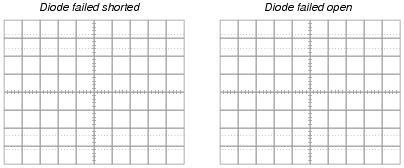• #### Question 4

How is it possible to determine the polarity of a rectifying diode (which terminal is the anode, and which terminal is the cathode) from its physical appearance?

• #### Question 5

The “1N400x” series of rectifying diodes are very popular for low-current applications. By “1N400x,” I mean the 1N4001, 1N4002, 1N4003, . . . 1N4007. Only one parameter differs between these different diode models. What parameter is this, and what is its significance?

• #### Question 6

Complete the following table of values for this diode circuit, assuming a typical forward voltage drop of 0.65 volts for the diode:• #### Question 7

Complete the following table of values for this diode circuit, assuming a typical forward voltage drop of 0.72 volts for the diode:• #### Question 8

Predict how all component voltages and currents in this circuit will be affected as a result of the following faults. Consider each fault independently (i.e. one at a time, no multiple faults):Diode D1 fails open:
Diode D1 fails shorted:
Resistor R1 fails open:
Solder bridge (short) past resistor R1:

For each of these conditions, explain why the resulting effects will occur.

• #### Question 9

Predict how all component voltages and currents in this circuit will be affected as a result of the following faults. Consider each fault independently (i.e. one at a time, no multiple faults):Diode D1 fails open:
Diode D1 fails shorted:
Resistor R1 fails open:
Resistor R2 fails open:

For each of these conditions, explain why the resulting effects will occur.

• #### Question 10

Predict how all component voltages and currents in this circuit will be affected as a result of the following faults. Consider each fault independently (i.e. one at a time, no multiple faults):Diode D1 fails open:
Diode D2 fails open:
Transformer T1 primary winding fails open:

For each of these conditions, explain why the resulting effects will occur.

• #### Question 11

An important parameter for many semiconductor components is thermal resistance, usually specified in units of degrees Celsius per Watt. What does this rating mean, and how is it related to temperature?

• #### Question 12

Rectifying diodes, like many other types of semiconductor components, should be derated at elevated ambient temperatures. Datasheets often provide “derating curves” that prescribe the maximum current for a range of ambient temperatures.

Explain just what “derating” is, and why it is so important for semiconductor devices.

• #### Question 13

Describe the operation of this diode testing circuit:Identify what the two light-emitting diodes (LEDs) will do when testing these three types of diodes:

Good diode
Diode failed shorted
Diode failed open
• #### Question 14

A useful piece of test equipment for semiconductor components is a curve tracer, used to produce current/voltage graphs for a component being tested. The graphs are typically displayed on an oscilloscope screen. Here is a very simple curve tracer circuit, designed to be used with an oscilloscope in X-Y mode:Describe what type of trace would be drawn by this circuit on an oscilloscope screen if a resistor was being tested. Then, show the trace for a normal rectifying diode.

• #### Question 15

Suppose we have an application where a DC generator provides power to charge a secondary-cell battery: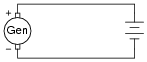The only problem with this setup is, the generator tries to act as a motor when the engine turning it is shut off, drawing power from the battery and discharging it. How could we use a rectifying diode to prevent this from happening?

• #### Question 16

What could you do if you had an application for a rectifying diode that required a forward current rating of 2.5 amps, but you only had model 1N4001 diodes available to use? How could you use multiple 1N4001 rectifying diodes to handle this much current?

• #### Question 17

Suppose you were building a simple half-wave rectifier circuit for a 480 volt AC source. The diode needs to withstand the full (peak) voltage of this AC source every other half-cycle of the waveform, or else it will fail. The bad news is, the only diodes you have available for building this rectifier circuit are model 1N4002 diodes.

Describe how you could use multiple 1N4002 rectifying diodes to handle this much reverse voltage.

• #### Question 18

What diode performance parameter establishes the limit for maximum frequency of AC which it may rectify? If you were to examine a diode datasheet, what parameter (or parameters) would be the most important in answering this question?

• #### Question 19

Find one or two real diodes and bring them with you to class for discussion. Identify as much information as you can about your diodes prior to discussion:

Polarity (which terminal is cathode and which is anode)
Forward voltage drop
Continuous current rating
Surge current rating
Continuous power rating